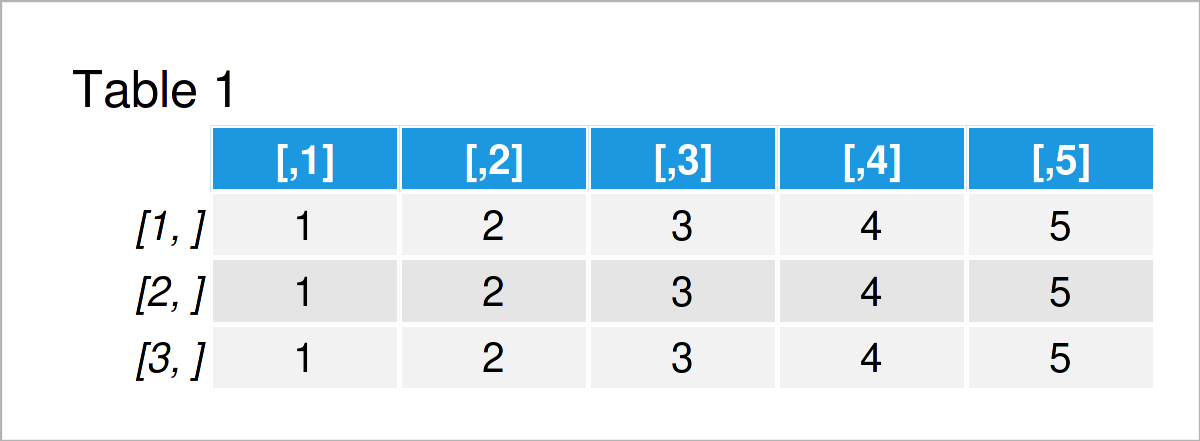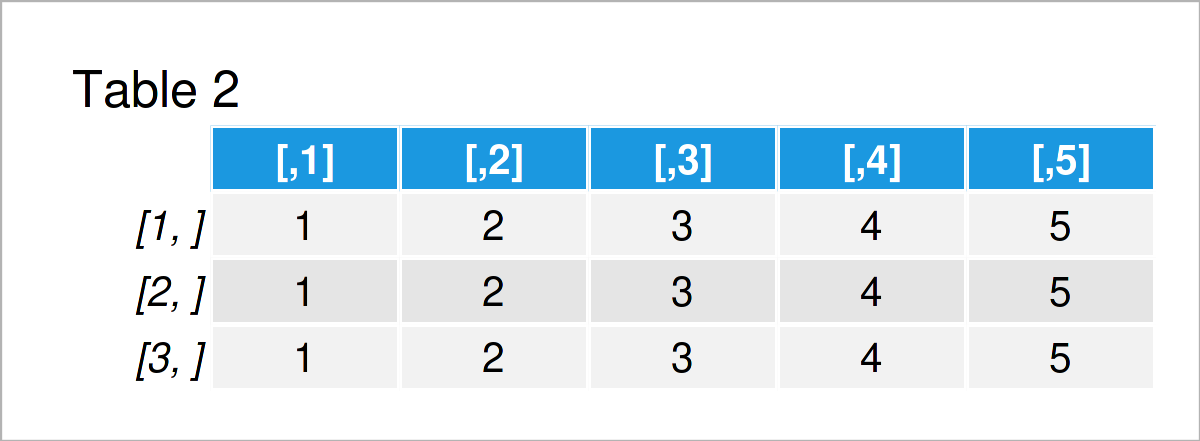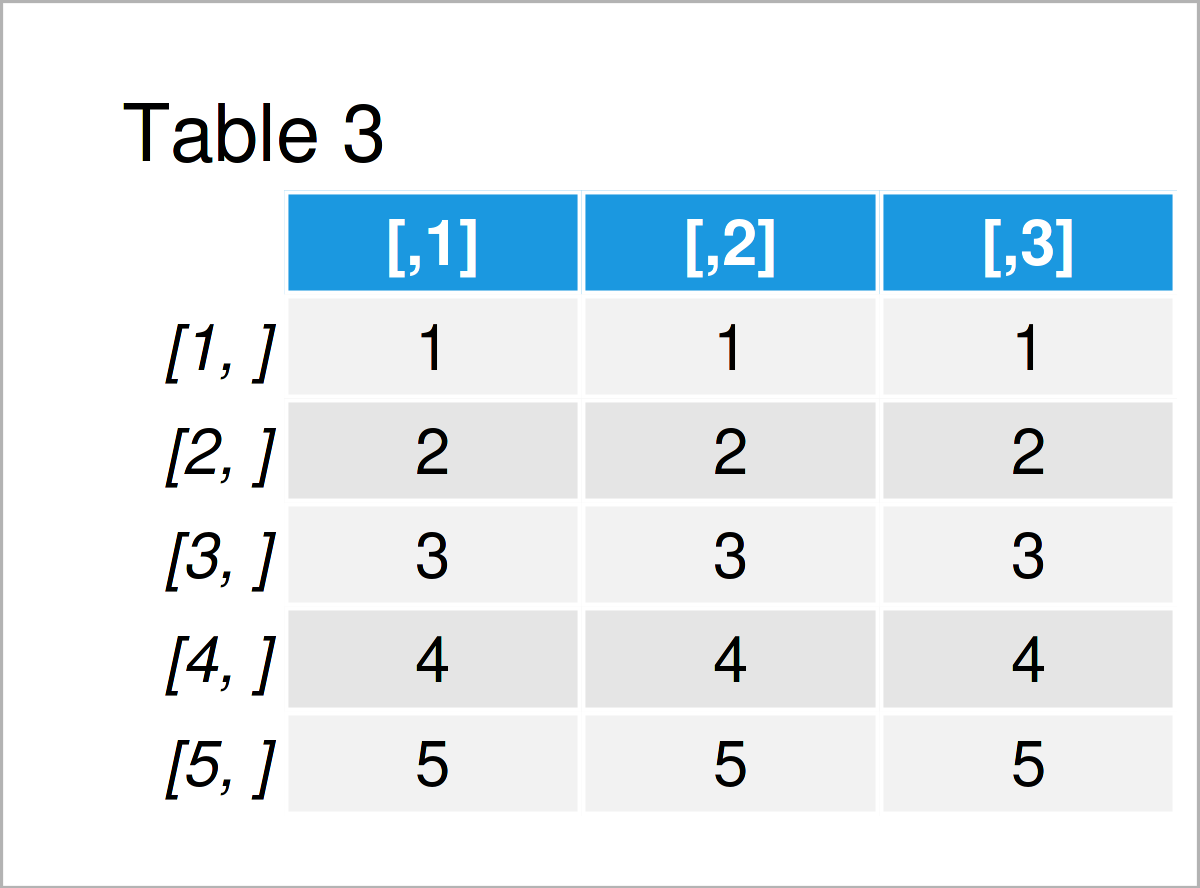# Replicate Vector in Matrix in R (3 Examples)

In this tutorial, I’ll show how to duplicate a vector in a matrix in the R programming language.

The content is structured as follows:

Let’s dive into it…

## Creation of Example Data

Have a look at the example data below:

```vec <- 1:5                      # Create example vector
vec                             # Print example vector
#  1 2 3 4 5```

As you can see based on the previous output of the RStudio console, our exemplifying vector contains five numeric elements.

## Example 1: Replicate Vector in Matrix Using t() & replicate() Functions

The following R programming code explains how to repeat a vector in the rows of a matrix multiple times.

For this task, we can apply the t() and replicate() functions as shown below:

```mat1 <- t(replicate(3, vec))    # Duplicate vector in matrix rows
mat1                            # Print matrix```The previous table illustrates the output of the R syntax above. As you can see, we have replicated our example vector three times.

## Example 2: Replicate Vector in Matrix Using matrix() Function

In Example 1, I have explained how to repeat a vector using the t() and replicate() functions.

This example shows how to use the matrix() function for this task.

Have a look at the following R programming code:

```mat2 <- matrix(vec,             # Duplicate vector in matrix rows
nrow = 3,
ncol = length(vec),
byrow = TRUE)
mat2                            # Print matrix```After executing the previous R programming code the matrix object illustrated in Table 2 has been created. It contains exactly the same values as the matrix created in Example 1. However, this time we have used the matrix() function to achieve this.

## Example 3: Replicate Vector as Columns in Matrix Using replicate() Function

The previous examples have explained how to replicate a vector object in the rows of a matrix.

In this example, I’ll demonstrate how to repeat a vector by columns.

To do this, we can use the replicate() function as shown below. Note that the following syntax is basically the same as in Example 1, but we are not using the t() function to transpose our data.

```mat3 <- replicate(3, vec)       # Duplicate vector in matrix columns
mat3                            # Print matrix```After executing the previous R programming code the matrix you can see in Table 3 has been created. As you can see, we have repeated our vector in three columns of our matrix.

## Video & Further Resources

Have a look at the following video on the Statistics Globe YouTube channel. In the video, I demonstrate the R code of this article.

Please accept YouTube cookies to play this video. By accepting you will be accessing content from YouTube, a service provided by an external third party.If you accept this notice, your choice will be saved and the page will refresh.

Additionally, you might have a look at the other tutorials on my homepage:

You have learned on this page how to replicate a vector in a matrix in R programming. Tell me about it in the comments section, if you have additional questions.

Subscribe to the Statistics Globe Newsletter